CBSE Class 10 Sample Paper for 2019 Boards

Class 10
Solutions of Sample Papers for Class 10 Boards

Question 28 (OR 1 st question)

The median of the following data is 525. Find the values of x and y if the total frequency is 100.

 Class Interval Frequency 0 – 100 2 100 – 200 5 200 – 300 x 300 – 400 12 400 – 500 17 500 – 600 20 600 – 700 y 700 – 800 9 800 – 900 7 900 – 100 4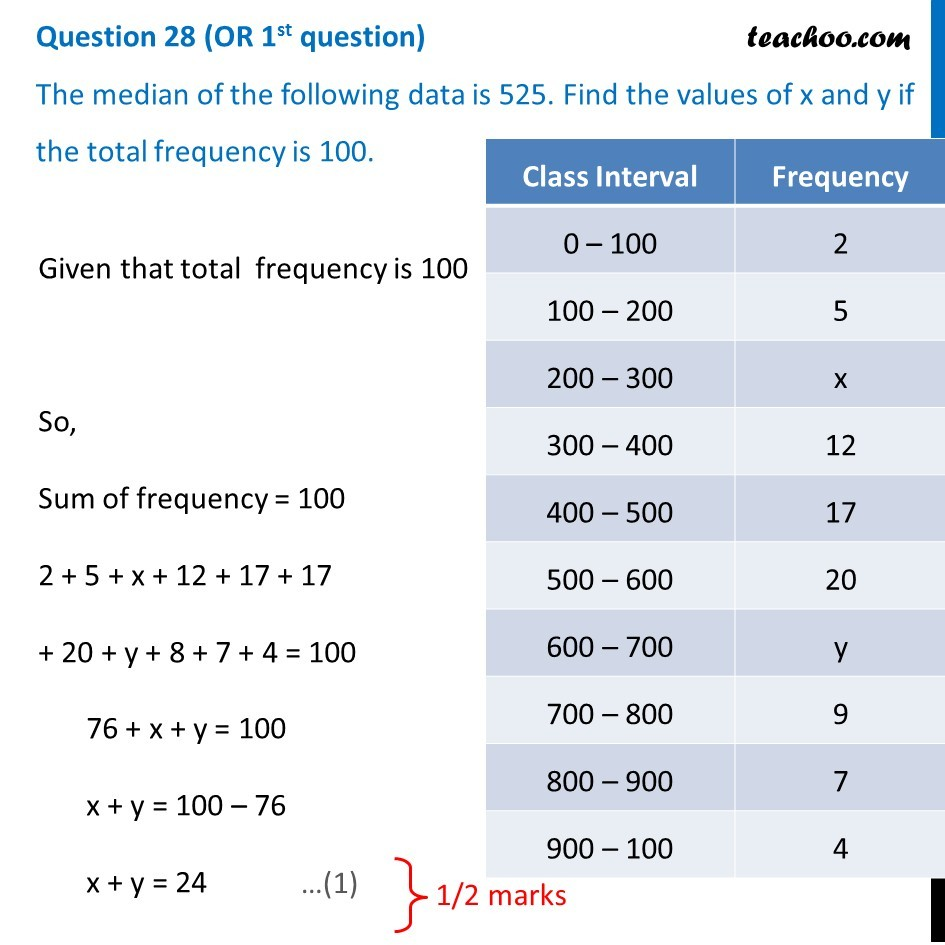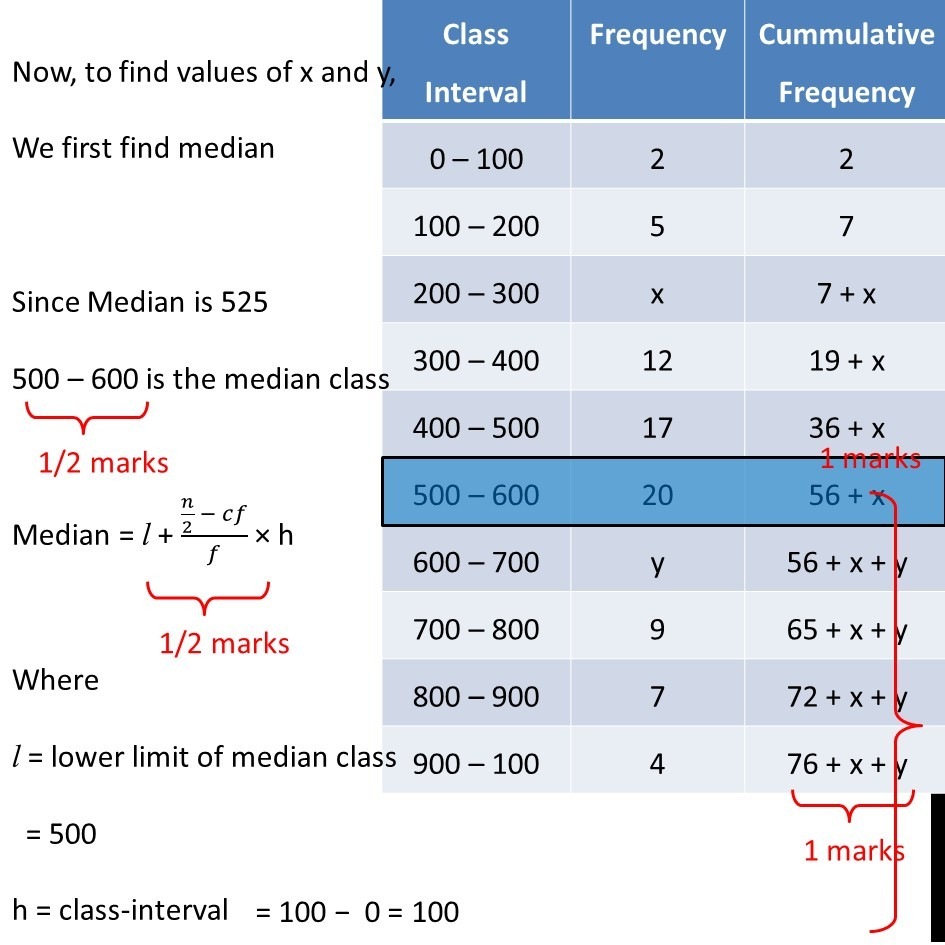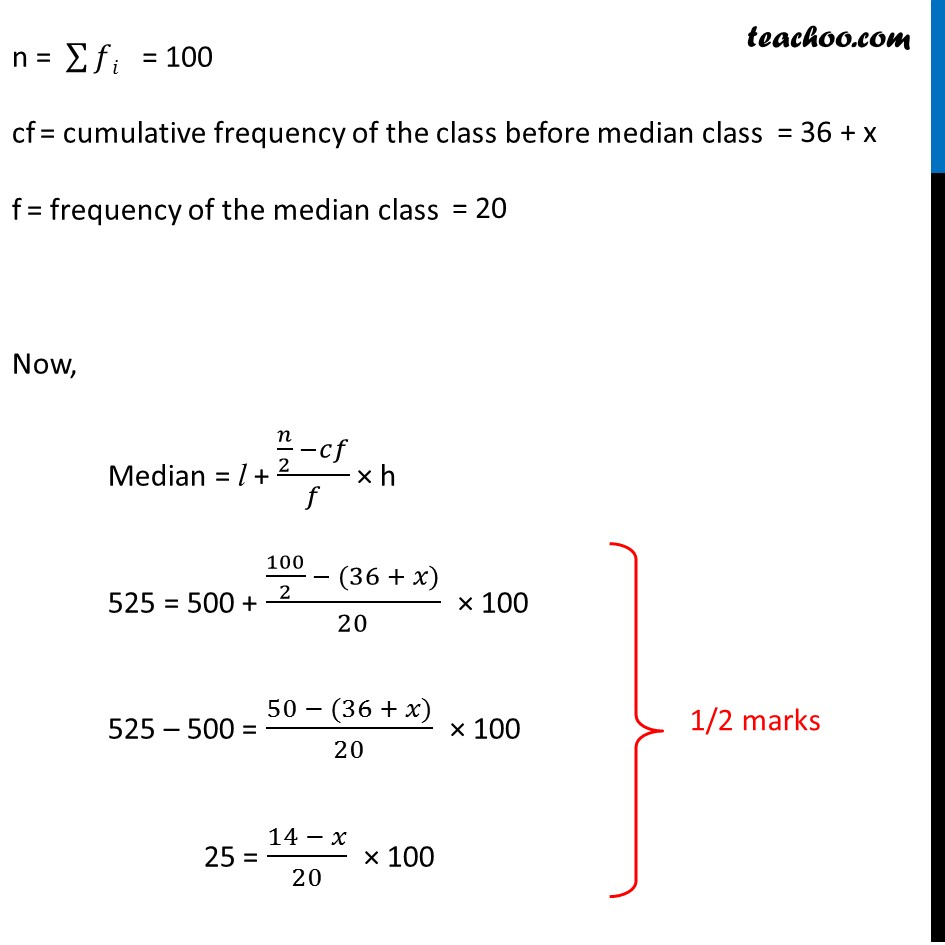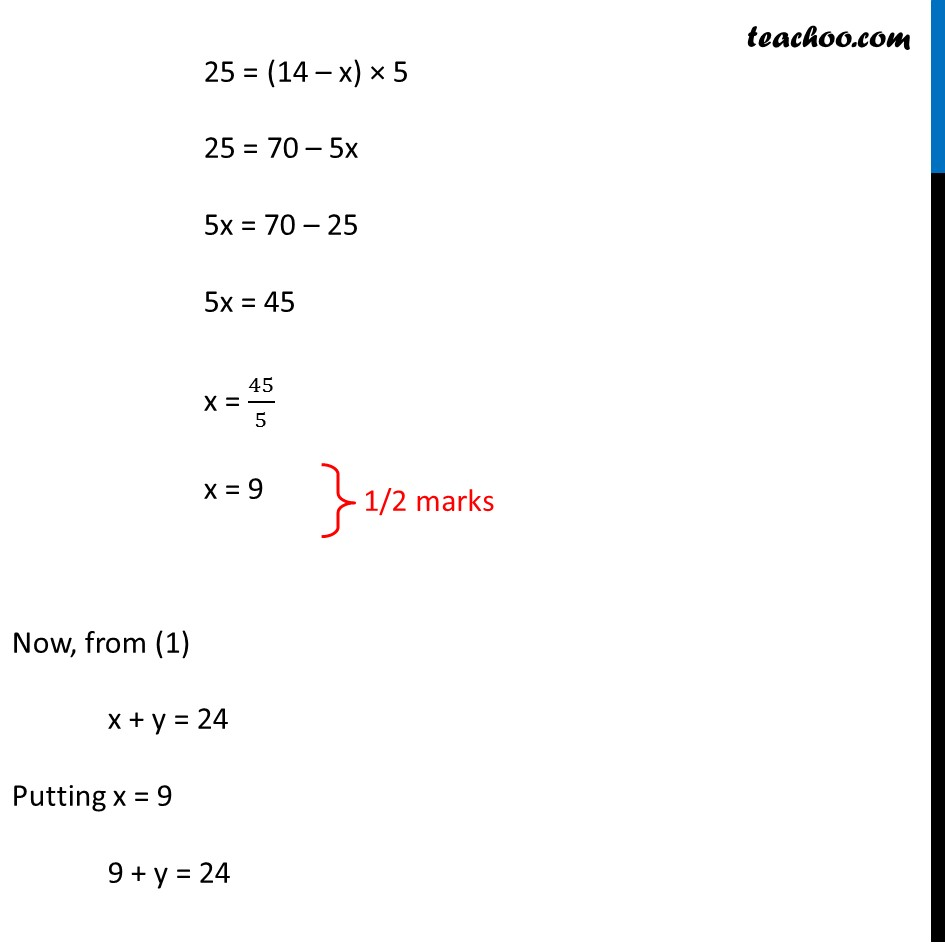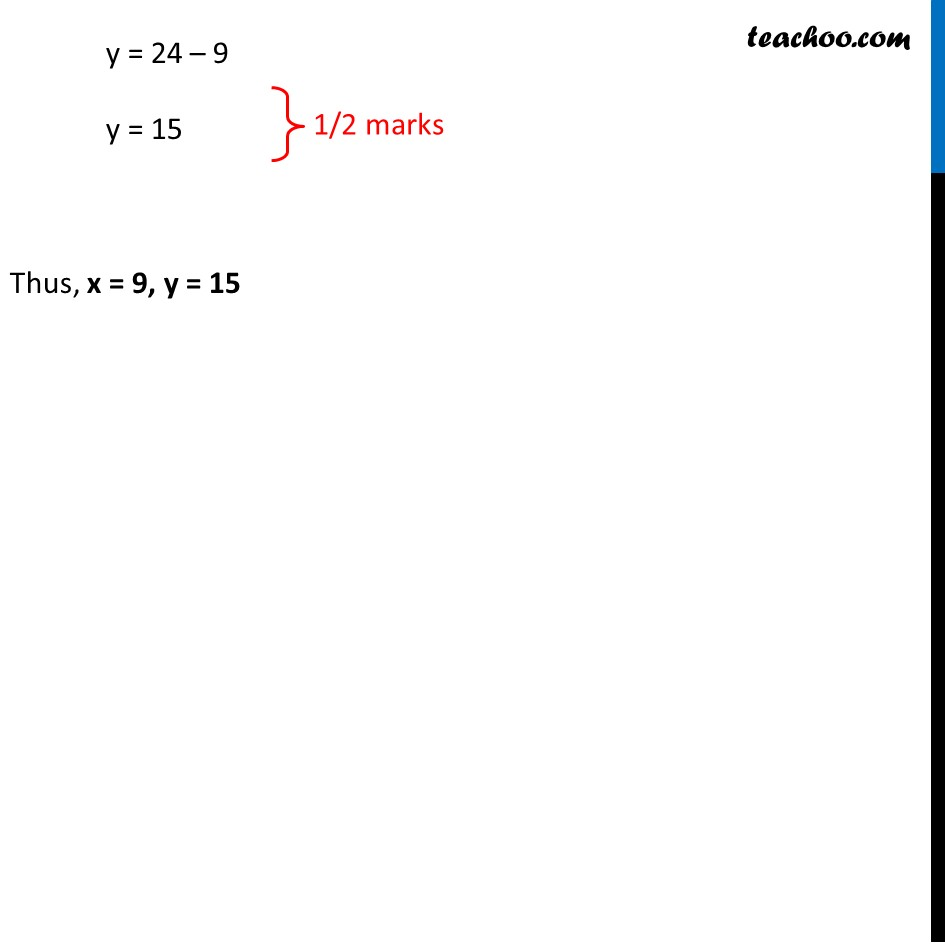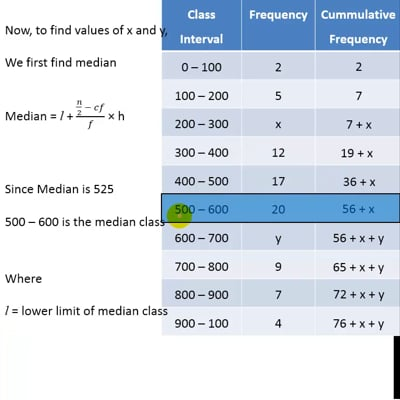This video is only available for Teachoo black users

Learn in your speed, with individual attention - Teachoo Maths 1-on-1 Class

### Transcript

Question 28 (OR 1st question) The median of the following data is 525. Find the values of x and y if the total frequency is 100. Given that total frequency is 100 So, Sum of frequency = 100 2 + 5 + x + 12 + 17 + 17 + 20 + y + 8 + 7 + 4 = 100 76 + x + y = 100 x + y = 100 – 76 x + y = 24 Now, to find values of x and y, We first find median Since Median is 525 500 – 600 is the median class Median = l + (𝑛/2 − 𝑐𝑓)/𝑓 × h Where l = lower limit of median class = 500 h = class-interval = 100 − 0 = 100 n = ∑▒𝑓𝑖 = 100 cf = cumulative frequency of the class before median class = 36 + x f = frequency of the median class = 20 Now, Median = l + (𝑛/2 −𝑐𝑓)/𝑓 × h 525 = 500 + (100/2 − (36 + 𝑥))/20 × 100 525 – 500 = (50 − (36 + 𝑥))/20 × 100 25 = (14 − 𝑥)/20 × 100 25 = (14 – x) × 5 25 = 70 – 5x 5x = 70 – 25 5x = 45 x = 45/9 x = 5 Now, from (1) x + y = 24 Putting x = 5 5 + y = 24 y = 24 – 5 y = 19 Thus, x = 5, y = 19